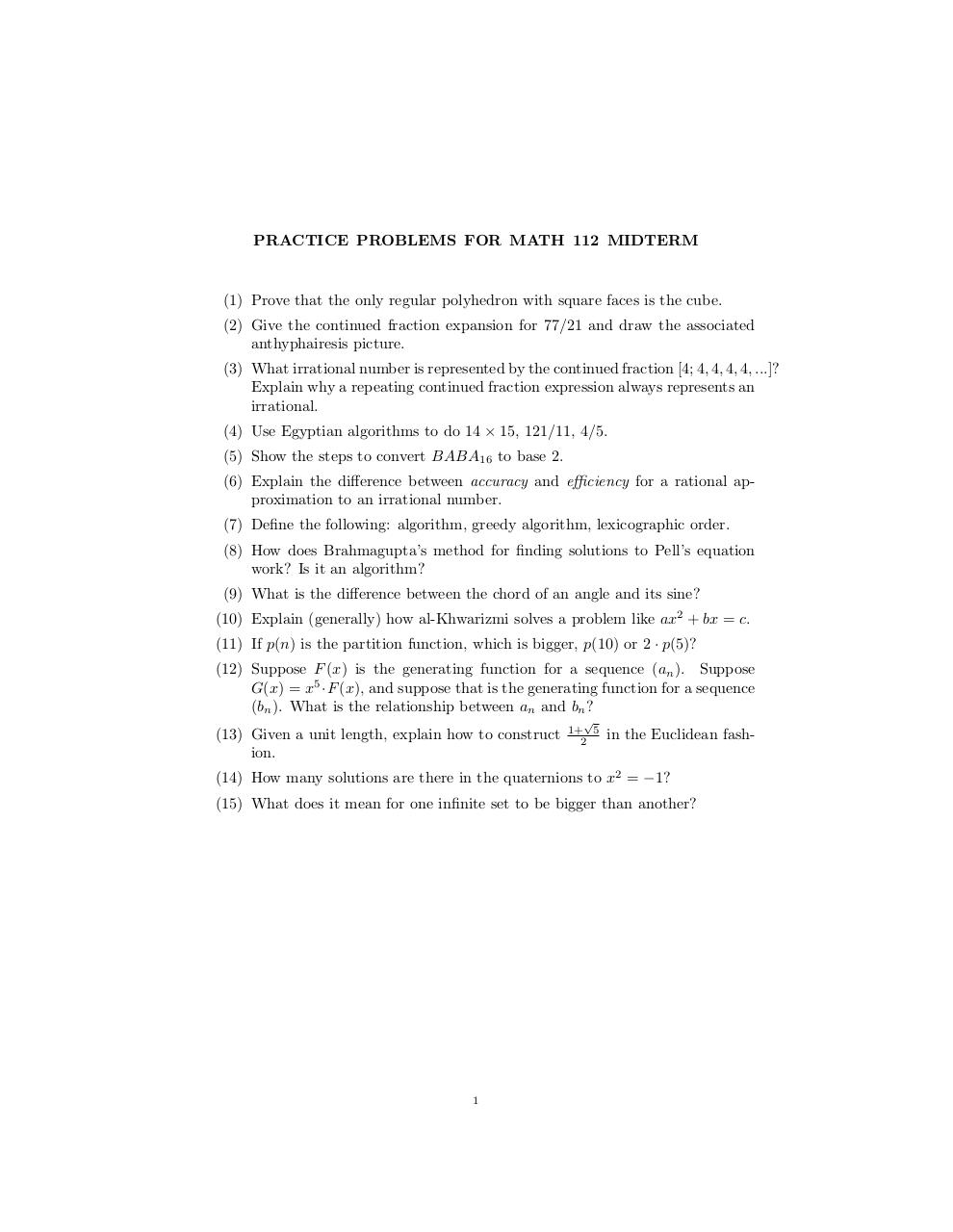# pracprob .pdf

### File information

Original filename: pracprob.pdf

This PDF 1.5 document has been generated by TeX / pdfTeX-1.40.17, and has been sent on pdf-archive.com on 05/04/2017 at 07:31, from IP address 130.64.x.x. The current document download page has been viewed 214 times.
File size: 83 KB (1 page).
Privacy: public file

pracprob.pdf (PDF, 83 KB)

### Document preview

PRACTICE PROBLEMS FOR MATH 112 MIDTERM

(1) Prove that the only regular polyhedron with square faces is the cube.
(2) Give the continued fraction expansion for 77/21 and draw the associated
anthyphairesis picture.
(3) What irrational number is represented by the continued fraction [4; 4, 4, 4, 4, ...]?
Explain why a repeating continued fraction expression always represents an
irrational.
(4) Use Egyptian algorithms to do 14 × 15, 121/11, 4/5.
(5) Show the steps to convert BABA16 to base 2.
(6) Explain the difference between accuracy and efficiency for a rational approximation to an irrational number.
(7) Define the following: algorithm, greedy algorithm, lexicographic order.
(8) How does Brahmagupta’s method for finding solutions to Pell’s equation
work? Is it an algorithm?
(9) What is the difference between the chord of an angle and its sine?
(10) Explain (generally) how al-Khwarizmi solves a problem like ax2 + bx = c.
(11) If p(n) is the partition function, which is bigger, p(10) or 2 · p(5)?
(12) Suppose F (x) is the generating function for a sequence (an ). Suppose
G(x) = x5 ·F (x), and suppose that is the generating function for a sequence
(bn ). What is the relationship between an and bn ?
(13) Given a unit length, explain how to construct
ion.

1+ 5
2

in the Euclidean fash-

(14) How many solutions are there in the quaternions to x2 = −1?
(15) What does it mean for one infinite set to be bigger than another?

1#### HTML Code

Copy the following HTML code to share your document on a Website or Blog

#### QR Code### Related keywords